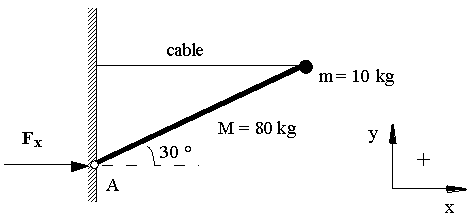# Flag pole finding horizontal force component at hinge.

• Parker Hays

## Homework Statement

A flag pole consists of a 80 kg rod of length L = 2 m with a 10 kg point mass attached to the end. The pole is hinged at the bottom and is tied to a horizontal cable as shown.
1. What is the x-component of F (Fx) exerted by the wall on the flag pole at the hinged point A?
(The picture shows the flag pole hinged at a 30 degree angle to the horizontal and the 10kg point mass is not on the hinged side).## Homework Equations

Net torque=0, Net force=0, τ=r x F=Iα.

## The Attempt at a Solution

I've attempted to find the location of the center of mass so that I can know where the gravity acts on the rod. I have gotten many different answers but none of them have been correct. The correct answer is 850N and I don't know how to get there, other than Fnet=0N and τnet=0Nm. I also think torque due to the hinge is zero since it is at the hinge and therefore r x F is zero.

#### Attachments

attempted to find the location of the center of mass
That is rarely necessary.
Just consider the torque that each force exerts (about some chosen axis) and sum them.
To choose an axis, look at the forces you do not know and do not need to find. If you can pick an axis on the intersection of their lines of action you will only need the one equation.
Otherwise you will need both torque and linear equations.

•CWatters